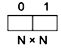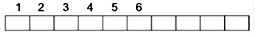# GATE Solved Paper 2017-19 - GATE 2018

• Option : A
• Explanation :
here \$ is higher precedence than # \$ is left associative because in the sub expression b \$ c \$ d, b \$ c will be evaluated first as per given tree.
As per the given tree structure right # if higher precedence than left #.
Hence it is right associative.

 Producer Consumer do{   wait(p);   wait(mutex);   //Add item to buffer   signal(mutex);   signal(Q); }while (1); do{   wait(R);   wait(mutex);   //Consume item from buffer   signal (mutex);   signal (); }while (1);
Which one of the following assignments to P, Q, R and S will yield the correct solution?

• A

P : full, Q: full, R: empty, S; empty• B

P: empty, Q: empty, R: full, S: full• C

P: full, Q: empty, R: empty, S: full• D

P: empty, Q: full, R: full, S: empty• Option : C
• Explanation :
Full = N, empty = 0, mutex = 1
Initially buffer will be empty, so consumer should not start first, so options B, D are eliminated.
With option A consumer will never consume the item, so it is wrong.
Option ‘C’ is the correct answer which proper functionality of produce and consumer.

• A

T1T2T3• B

T1T1T3• C

T2T1T3• D

T3T3• Option : D
• Explanation : T3T3 because from first T3 bbaac is taken from second T3 abc is taken longest possible prefix. Hence T3T3 token output.

• A

None of (i), (ii), (iii), (iv) can be exactly represented.• B

Only (ii) cannot be exactly represented.• C

Only (iii) and (iv) cannot be exactly represented.• D

Only (i) and (ii) cannot be exactly represented.• Option : C
• Explanation :
Binary code: (b7b6b5b4b3 ⋅ b2b1b0)2
(31.5)10 = (11111.1)2
(0.875)10 = (0.111)2
(12.100)10 = (01100.000110.....)2
↓
Only 3 bits of fraction
space available
So can't be stored.
(3.001)10 = (00011.000000..........)2
It is also not accurate storage.

• A

Q and S only• B

P and S only• C

P and R only• D

P, Q and S only• Option : D
• Explanation :
P : Set of rational number → countable
Q : Set of functions from {0, 1} to N → N0 can be assigned in N ways
1 can be assigned in N ways
There are functions, cross product of countable set in countable.
R : Set of functions from N to {0, 1}Each of thus boxes can be assigned to 0 or 1 so each such function is a binary number with infinite number of bits.
Related Quiz.
GATE 2018